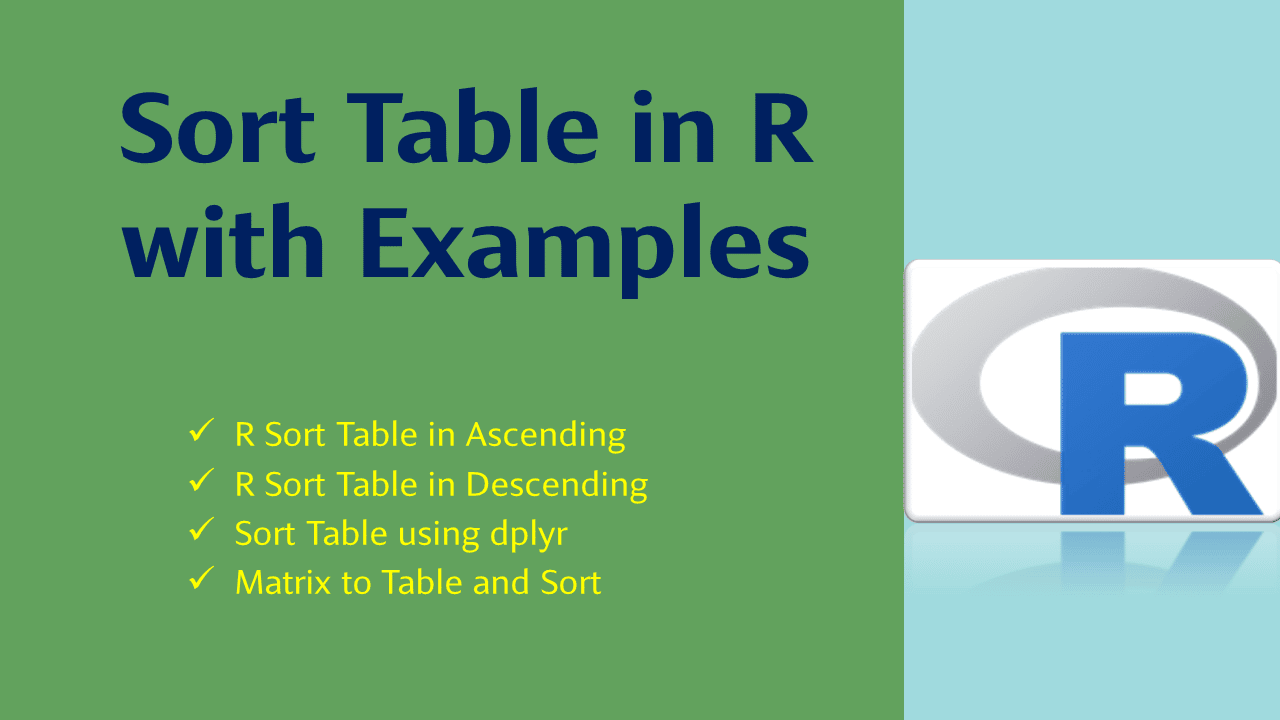# Sort Table in R with Examples

In this article, I will explain how to sort or order a table by Frequency (Freq) in R. Table in R is used to represent the data with the column/variable name and the frequency of how many times each is repeated. By using order() and arrange() functions we can sort the table by ascending order, and descending order in R.

## 1. Quick Examples of Sorting Table

Following are quick examples of how to sort tables.

``````
#  Create Vector with repeated values
vec <- c(letters[5:12], letters[7:10],  letters[9:10])
vec

# Convert vector to table
tab <- table(vec)

# Sort table in ascending order
sorted_tab <- tab[order(tab)]
sorted_tab

# Sort table in descending order
sorted_tab <- tab[order(tab,decreasing = TRUE)]
sorted_tab

# Using dplyr
sorted_tab <- tab %>%
as.data.frame() %>%
arrange(desc(Freq))
sorted_tab
``````

First, let’s create a character vector in R and convert it to the table by using table() function.

``````
# Create Vector with repeated values
vec <- c(letters[5:12], letters[7:10],  letters[9:10])
vec

# Convert vector to table
tab <- table(vec)
``````

Yields below vector

And the below R table. Note that the table contains the value and the frequency of the value (how many times each value is repeated).

## 2. R Sort Table in Ascending

By using R base function order() we can sort the table in ascending order. This function takes the table indices, so we have to use [] – index and inside this, we can apply the `order()` function.

``````
# Sort table in ascending order
sorted_tab <- tab[order(tab)]
sorted_tab
``````

Yields below output.

## 3. R Sort Table in Descending

Similarly, you can also use R base order() function along with `decreasing = TRUE` param to sort the table in descending order.

``````
# Sort table in descending order
sorted_tab <- tab[order(tab,decreasing = TRUE)]
sorted_tab
``````

Yields below output.

## 4. Sort Table using dplyr

Another way to order the table in R is by converting the table into DataFrame and using the dplyr arrange() function to sort the dataframe.

The arrange() function from dplyr package is also used to arrange the values in an ascending or descending order. To use arrange() function, you have to install dplyr first using install.packages(‘dplyr’) and load it using `library(dplyr)`.

All functions in dplyr package take `data.frame` as a first argument. When we use `dplyr` package, we mostly use the infix operator `%>%` from `magrittr`, it passes the left-hand side of the operator to the first argument of the right-hand side of the operator. For example, `x %>% f(y)` converted into `f(x, y)` so the result from the left-hand side is then “piped” into the right-hand side.

``````
# Using dplyr
sorted_tab <- tab %>%
as.data.frame() %>%
arrange(desc(Freq))
sorted_tab
``````

Yields below output.

## 5. Matrix to Table and Sort

Let’s take a little complex example and see how to sort the table in R.

``````
# Create table
tab <- matrix(c(6, 3, 2, 9, 6, 34, 26, 4, 5), ncol=3, byrow=TRUE)
colnames(tab) <- c('col1','col2','col3')
rownames(tab) <- c('row1','row2','row3')
tab <- as.table(tab)
tab
``````

Yields below output.

Now sort the table by Frequency `Freq` column.

``````
# Sort table by converting to DataFrame
tab2 <- tab %>%
as.data.frame() %>%
arrange(desc(Freq))
tab2
``````

I will leave this to you to run and explore the output.

## Conclusion

In this R article, you have learned how to sort/order the table by using the order() and arrange() function. By using these functions you have also learned to sort tables in ascending or descending order.

## References

### Naveen (NNK)

Naveen (NNK) is a Data Engineer with 20+ years of experience in transforming data into actionable insights. Over the years, He has honed his expertise in designing, implementing, and maintaining data pipelines with frameworks like Apache Spark, PySpark, Pandas, R, Hive and Machine Learning. Naveen journey in the field of data engineering has been a continuous learning, innovation, and a strong commitment to data integrity. In this blog, he shares his experiences with the data as he come across. Follow Naveen @ @ LinkedIn